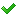•闪存
•博客
• 发言小组
• 投递新闻
• 提问博问
• 添加收藏
•文库

7

##100新手一枚，求各位大神帮帮忙，我用什么更好的解决方法可以不用if判断

1=高级返佣，2=中级返佣，3=初级返佣 D=返佣等级 Y=营业部 Z=中级 C=初级 以上是代码中的字符代表的意义 if(Y.D=3) { if(Z.D=3) { if(C.D=3) { } if(
3

8